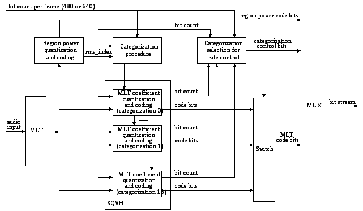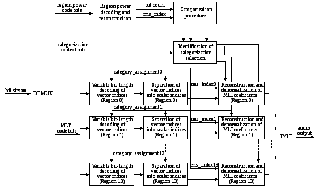# ITU-T G.722.1 and G.722.1 Annex C

 Page 11/16 Date 23.04.2018 Size 1 Mb. #46252

## ITU-T G.722.1 and G.722.1 Annex C

The main body of [ITU-T G.722.1] describes a wideband coding algorithm that provides an audio bandwidth of 50 Hz to 7 kHz, operating at a bit rate of 24 kbit/s or 32 kbit/s. Annex C of [ITU-T G.722.1] is a doubled form of the G.722.1 main body to permit 14 kHz audio bandwidth using a 32 kHz audio sample rate, at 24, 32, and 48 kbit/s. Both G.722.1 and G.722.1 Annex C codecs feature very high audio quality, extremely low computational complexity, and low algorithmic delay compared to other state-of-the-art audio coding algorithms.

The G.722.1 algorithm is based on transform coding, using a Modulated Lapped Transform (MLT) and operates on frames of 20 ms corresponding to 320 samples at a 16 kHz sampling rate. Because the transform window length is 640 samples and a 50 percent overlap is used between frames, the effective look-ahead buffer size is 320 samples. Hence the total algorithmic delay of the coder is 40 ms. Figure 7-5 shows a block diagram of the encoder.Figure 7-5: Block diagram of the G.722.1 encoder

The MLT performs a frequency spectrum analysis on audio samples and converts the samples from the time domain into a frequency domain representation. Every 20 ms the most recent 640 audio samples are fed to the MLT and transformed into a frame of 320 transform coefficients centred at 25 Hz intervals. In each frame the MLT transform coefficients are divided into 16 regions, each having 20 transform coefficients and representing a bandwidth of 500 Hz. As the bandwidth is 7 kHz, only the 14 lowest regions are used. For each region the region power or the root-mean-square (rms) value of the MLT transform coefficients in the region is computed and scalar quantized with a logarithmic quantizer The obtained quantization indices are differentially coded and then Huffman coded with a variable number of bits. Using the quantized region power indices and the number of bits remaining in the frame, the categorization procedure generates 16 possible categorizations to determine the parameters used to quantize and code the MLT transform coefficients. Then, the MLT transform coefficients are normalized, scalar quantized, combined into vectors, and Huffman coded. The bit stream is transmitted on the channel in 3 parts: region power code bits, 4 categorization control bits, and then code bits for MLT transform coefficients.

G.722.1 Annex C has the same algorithmic steps as the G.722.1 main body, except that the algorithm is doubled to accommodate the 14 kHz audio bandwidth. G.722.1 Annex C still operates on frames of 20 ms and has an algorithmic delay of 40 ms, but due to the higher sampling frequency the frame length is doubled to 640 samples from 320 samples and the transform window size increases to 1280 samples from 640 samples. Compared to the G.722.1 main body, the specific differences in the G.722.1 Annex C encoder are as follows:

• Double the MLT transform length from 320 to 640 samples

• Double the number of frequency regions from 14 to 28

• Double the sizes of Huffman coding tables for encoding quantized region power indices

• Double the threshold for adjusting the number of available bits from 320 to 640Figure 7-6: Block diagram of the G.722.1 decoder

Figure 7-6 shows a block diagram of the G.722.1 decoder. In each frame the region power code bits are first extracted from the received data and then decoded to obtain the quantization indices for the region powers. Using the same categorization procedure as the encoder, the set of 16 possible categorizations computed by the encoder are recovered and the categorization used to encode MLT transform coefficients is found with the received four categorization control bits. For each region the variable bit-length codes for MLT transform coefficients are decoded with the appropriate category and the MLT transform coefficients are reconstructed. The reconstructed MLT transform coefficients are converted into time domain audio samples by an Inverse MLT (IMLT). Each IMLT operation takes in 320 MLT transform coefficients to produce 320 audio samples.

The following are the main changes in the G.722.1Annex C decoder when compared to G.722.1.

• Double the number of frequency regions from 14 to 28

• Double the threshold for adjusting the number of available bits from 320 to 640

• Extend the centroid table used for reconstruction of MLT transform coefficients

• Double the IMLT transform length from 320 to 640 samples

Low complexity is a major technical advantage of G.722.1 and G.722.1 Annex C compared to other codecs with similar performance in this bit-rate range. Table 7-1 presents the computational complexity in units of Weighted Million Operations per Second (WMOPS)  and memory requirements in bytes of G.722.1 and G.722.1 Annex C, respectively.

Table 7-1: Computational complexity and memory requirements

 Codec Bit rate (kbit/s) Encoder (WMOPS) Decoder (WMOPS) Encoder + Decoder (WMOPS) RAM (bytes) Data-ROM (bytes) G.722.1 24 2.3 2.7 5.0 11 K 20 K 32 2.4 2.9 5.3 G.722.1 Annex C 24 4.5 5.3 9.7 18 K 30 K 32 4.8 5.5 10.3 48 5.1 5.9 10.9

In March 2005, as a part of the G.722.1 Annex C development process in ITU-T, subjective characterization tests were performed on G.722.1 Annex C by an independent listening lab according to a test plan designed by the ITU-T Q7/SG12 Speech Quality Experts Group (SQEG. A well-known MPEG audio codec was used as the reference codec in the tests. Statistical analysis of the test results showed that G.722.1 Annex C met all performance requirements. For speech signals, G.722.1 Annex C was better than the reference codec at 24 and 32 kbit/s and G.722.1 Annex C at 48 kbit/s was not worse than the reference codec operating at either 48 or 64 kbit/s. For music and mixed content such as film trailers, news, jingles and advertisement, G.722.1 Annex C was better than the reference codec at all bit rates and G.722.1 Annex C at 48 kbit/s was also better than the reference codec operating at 64 kbit/s .

The RTP payload for G.722.1 and G.722.1 Annex C is specified in ITU-T Recommendation G.722.1 Annex A, and also specified in [IETF RFC 3047] and [IETF RFC 5577] which supersedes RFC 3047 and adds support for G.722.1 Annex C.

ANSI-C source code reference implementations of both encoder and decoder parts if G.722.1 and G.722.1 Annex C are available as an integral part of [ITU-T G.722.1] for both fixed-point and floating-point arithmetic.